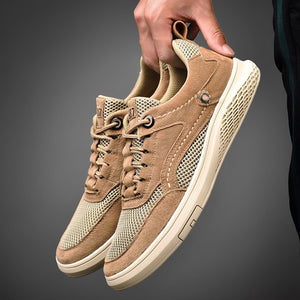# Men fashion Genuine Leather Loafers

• \$49.95 USD
Unit price per
• Save \$40.00 USD

***Size chart***

EU Size36=US5     =Foot Length 23.0cm
EU Size37=US5.5   =Foot Length 23.5cm
EU Size38=US6      =Foot Length 24.0cm
EU Size39=US6.5   =Foot Length 24.5cm
EU Size40=US7      =Foot Length 25.0cm
EU Size41=US8      =Foot Length 25.5cm
EU Size42=US8.5   =Foot Length 26.0cm
EU Size43=US9.5   =Foot Length 26.5cm
EU Size44=US10    =Foot Length 27.0cm
EU Size45=US11    =Foot Length 27.5cm
EU Size46=US12    =Foot Length 28.0cm
EU Size47=US12.5 =Foot Length 28.5cm